Скачать презентацию A new approach to introductory statistics Nathan Tintle

a98dd9e9e23d6cf87288b1b8138c8b4e.ppt

• Количество слайдов: 23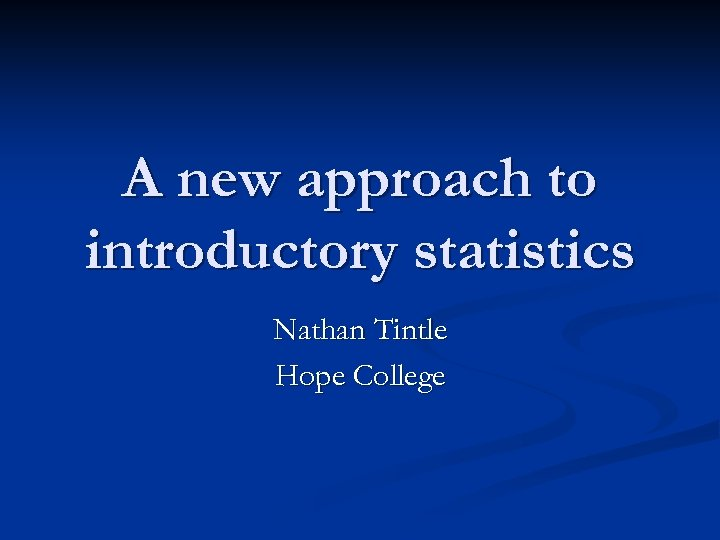A new approach to introductory statistics Nathan Tintle Hope College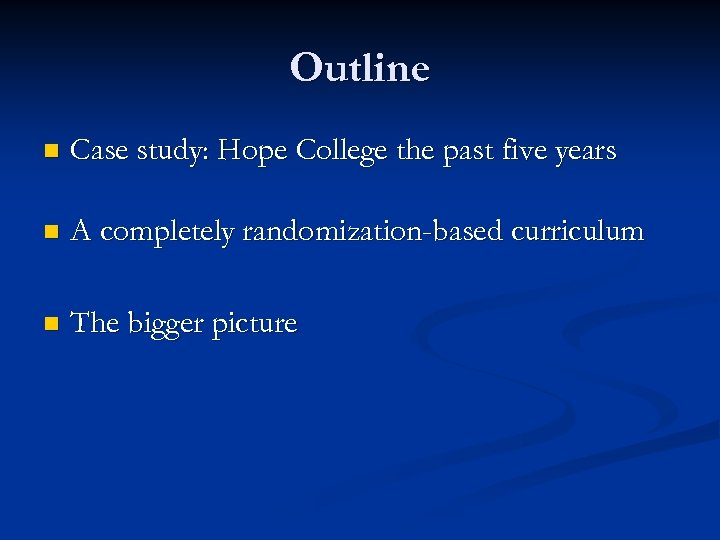Outline n Case study: Hope College the past five years n A completely randomization-based curriculum n The bigger picture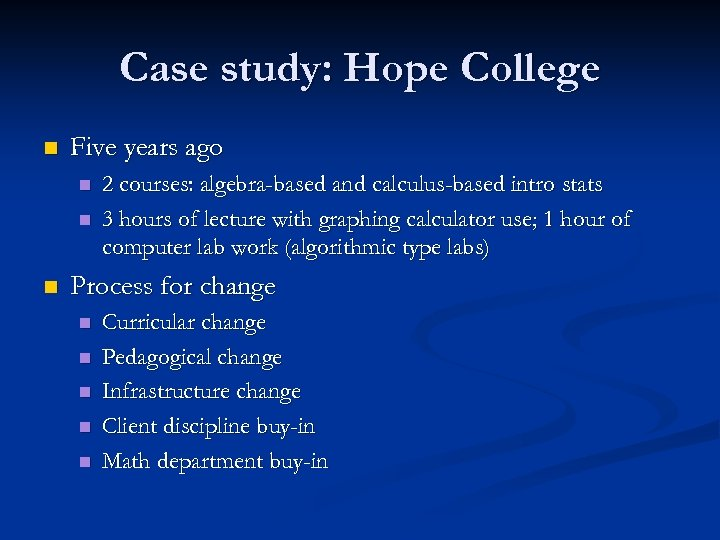Case study: Hope College n Five years ago n n n 2 courses: algebra-based and calculus-based intro stats 3 hours of lecture with graphing calculator use; 1 hour of computer lab work (algorithmic type labs) Process for change n n n Curricular change Pedagogical change Infrastructure change Client discipline buy-in Math department buy-in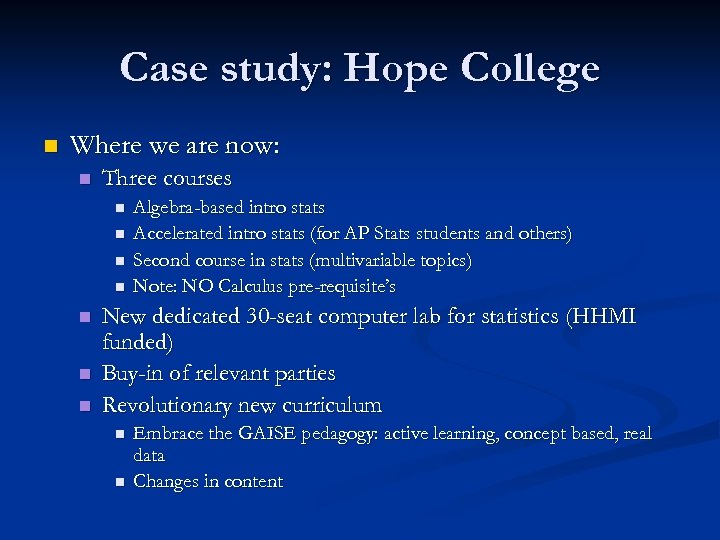Case study: Hope College n Where we are now: n Three courses n n n n Algebra-based intro stats Accelerated intro stats (for AP Stats students and others) Second course in stats (multivariable topics) Note: NO Calculus pre-requisite’s New dedicated 30 -seat computer lab for statistics (HHMI funded) Buy-in of relevant parties Revolutionary new curriculum n n Embrace the GAISE pedagogy: active learning, concept based, real data Changes in content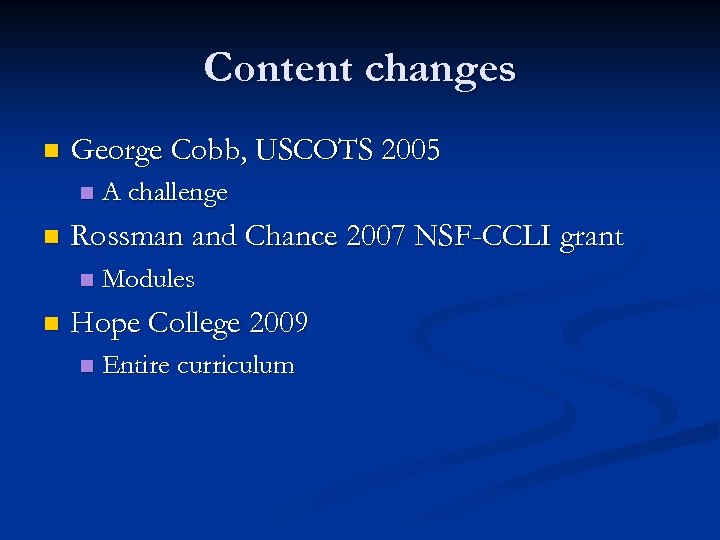Content changes n George Cobb, USCOTS 2005 n n Rossman and Chance 2007 NSF-CCLI grant n n A challenge Modules Hope College 2009 n Entire curriculum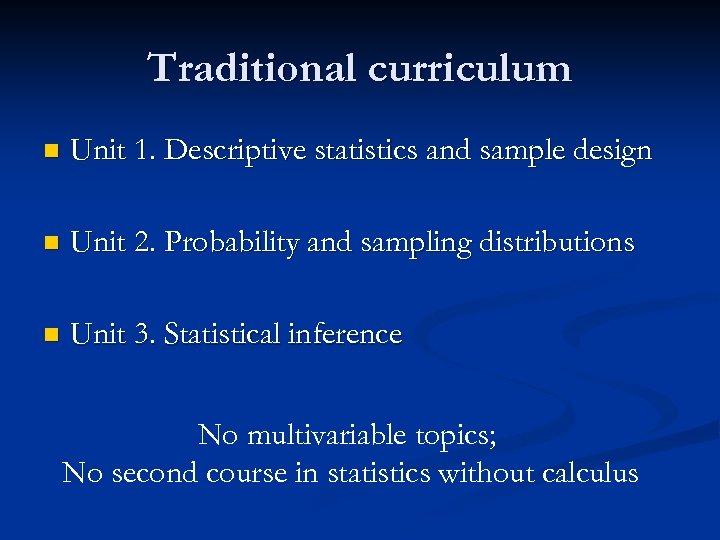Traditional curriculum n Unit 1. Descriptive statistics and sample design n Unit 2. Probability and sampling distributions n Unit 3. Statistical inference No multivariable topics; No second course in statistics without calculus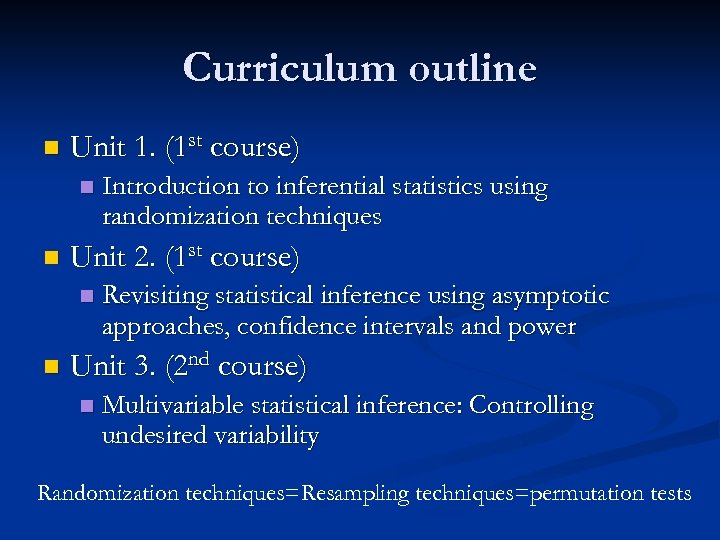Curriculum outline n Unit 1. (1 st course) n n Unit 2. (1 st course) n n Introduction to inferential statistics using randomization techniques Revisiting statistical inference using asymptotic approaches, confidence intervals and power Unit 3. (2 nd course) n Multivariable statistical inference: Controlling undesired variability Randomization techniques=Resampling techniques=permutation tests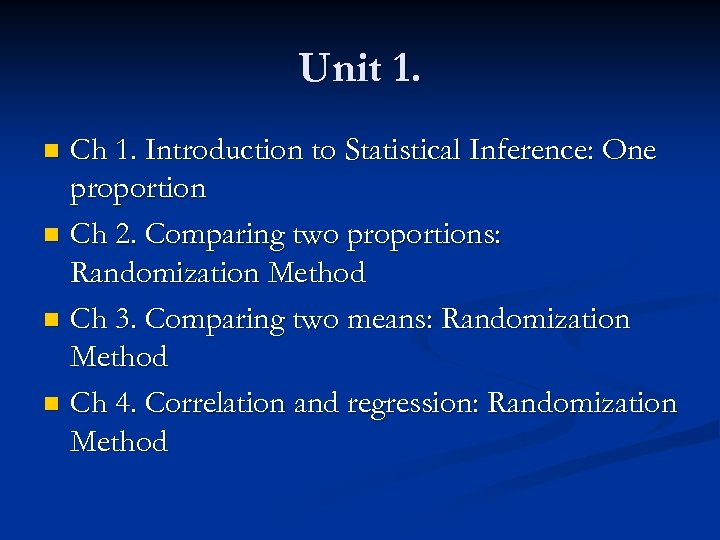Unit 1. Ch 1. Introduction to Statistical Inference: One proportion n Ch 2. Comparing two proportions: Randomization Method n Ch 3. Comparing two means: Randomization Method n Ch 4. Correlation and regression: Randomization Method n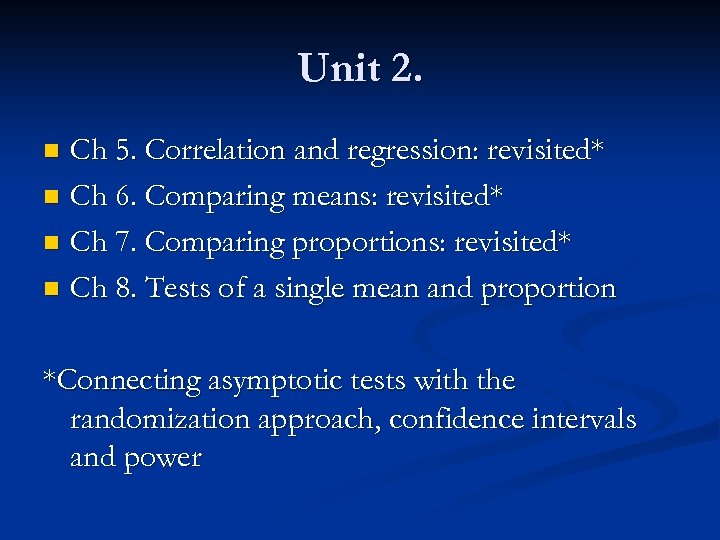Unit 2. Ch 5. Correlation and regression: revisited* n Ch 6. Comparing means: revisited* n Ch 7. Comparing proportions: revisited* n Ch 8. Tests of a single mean and proportion n *Connecting asymptotic tests with the randomization approach, confidence intervals and power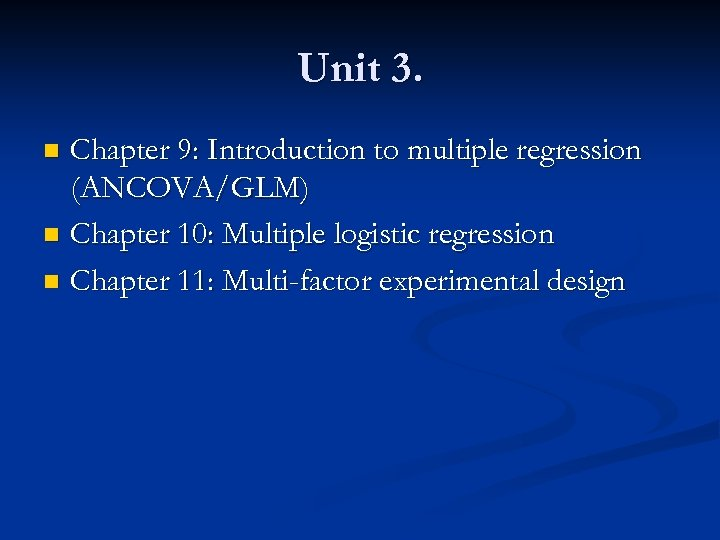Unit 3. Chapter 9: Introduction to multiple regression (ANCOVA/GLM) n Chapter 10: Multiple logistic regression n Chapter 11: Multi-factor experimental design n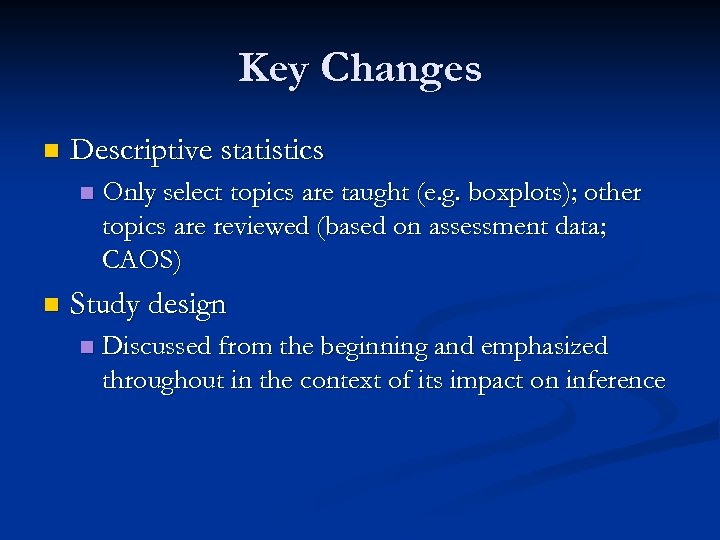Key Changes n Descriptive statistics n n Only select topics are taught (e. g. boxplots); other topics are reviewed (based on assessment data; CAOS) Study design n Discussed from the beginning and emphasized throughout in the context of its impact on inference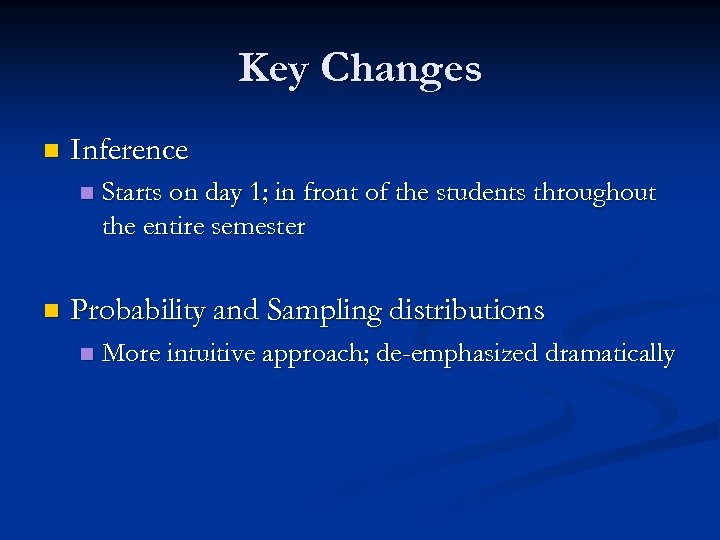Key Changes n Inference n n Starts on day 1; in front of the students throughout the entire semester Probability and Sampling distributions n More intuitive approach; de-emphasized dramatically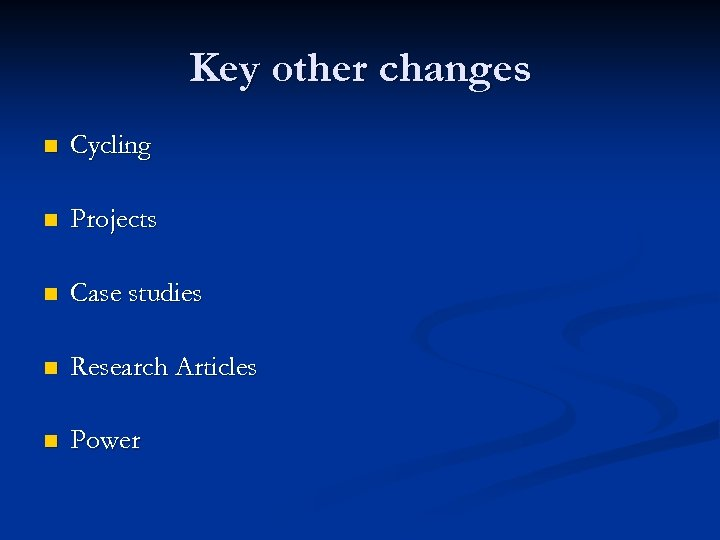Key other changes n Cycling n Projects n Case studies n Research Articles n Power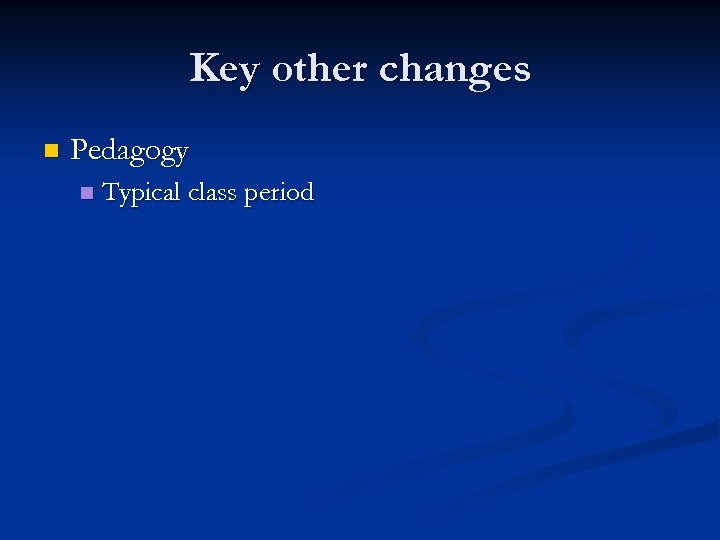Key other changes n Pedagogy n Typical class period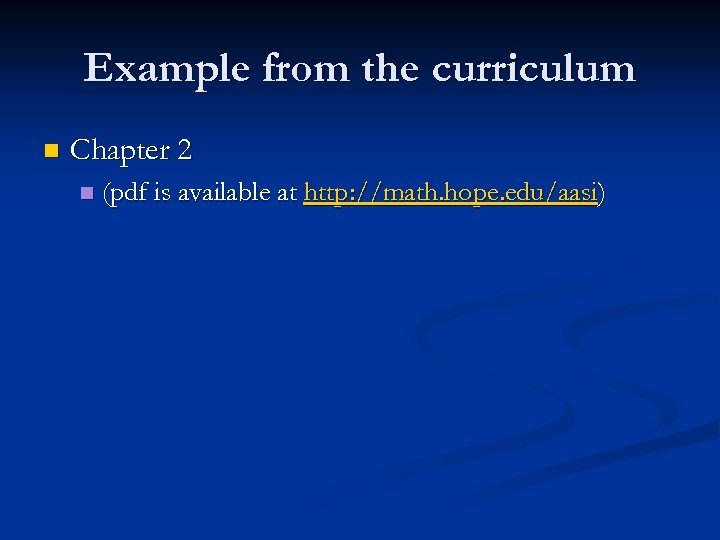Example from the curriculum n Chapter 2 n (pdf is available at http: //math. hope. edu/aasi)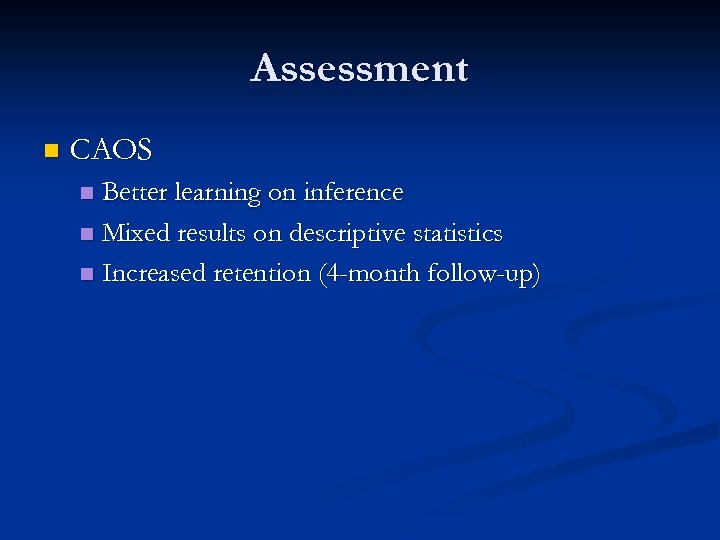Assessment n CAOS Better learning on inference n Mixed results on descriptive statistics n Increased retention (4 -month follow-up) n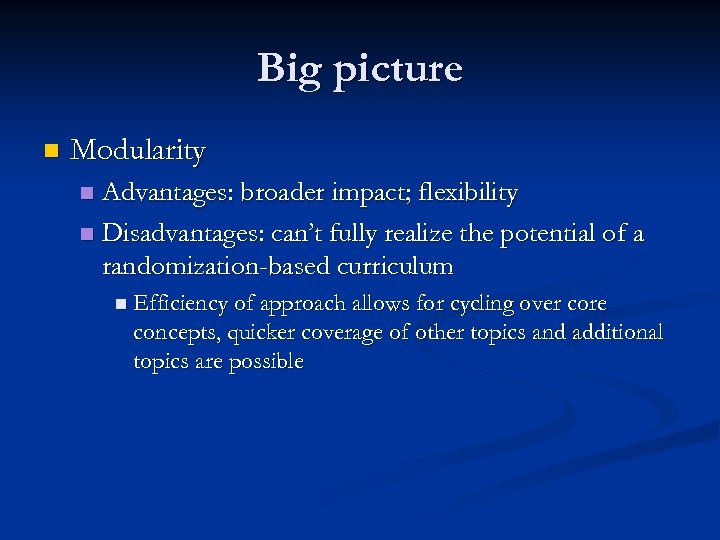Big picture n Modularity Advantages: broader impact; flexibility n Disadvantages: can’t fully realize the potential of a randomization-based curriculum n n Efficiency of approach allows for cycling over core concepts, quicker coverage of other topics and additional topics are possible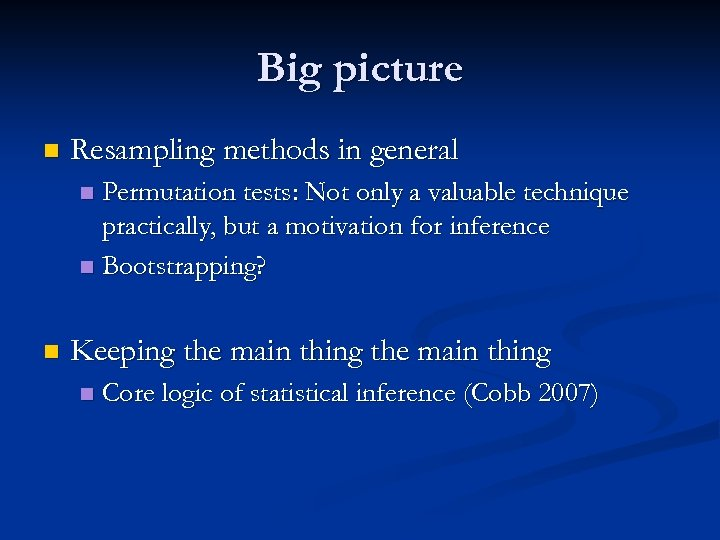Big picture n Resampling methods in general Permutation tests: Not only a valuable technique practically, but a motivation for inference n Bootstrapping? n n Keeping the main thing n Core logic of statistical inference (Cobb 2007)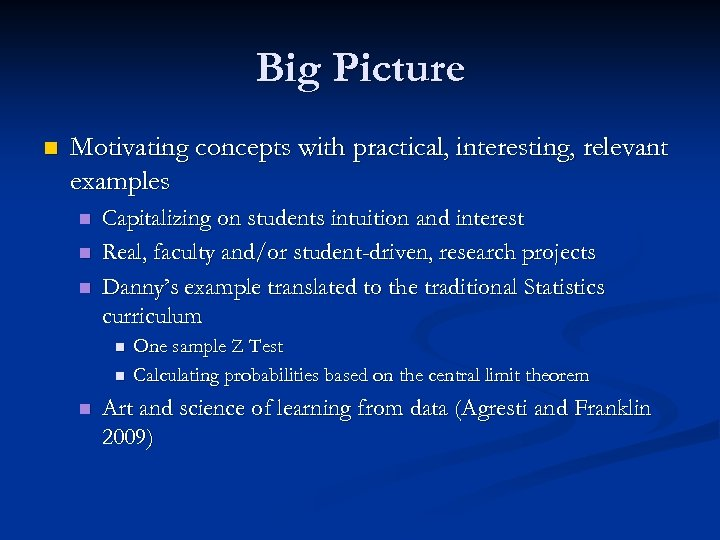Big Picture n Motivating concepts with practical, interesting, relevant examples n n n Capitalizing on students intuition and interest Real, faculty and/or student-driven, research projects Danny’s example translated to the traditional Statistics curriculum n n n One sample Z Test Calculating probabilities based on the central limit theorem Art and science of learning from data (Agresti and Franklin 2009)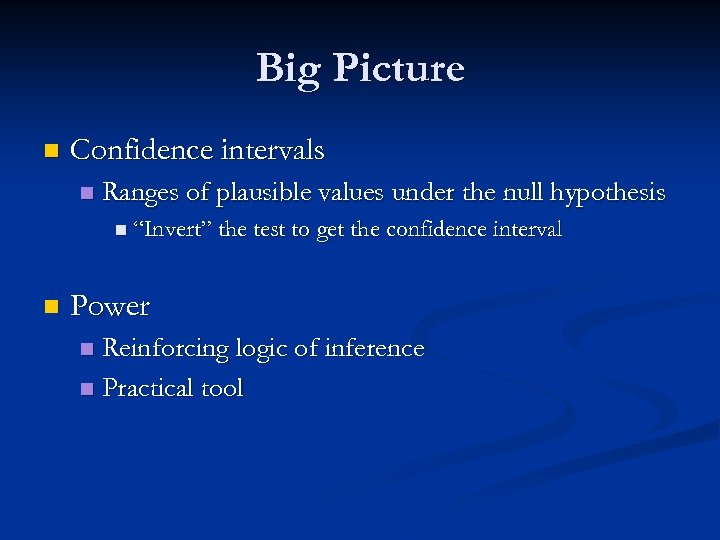Big Picture n Confidence intervals n Ranges of plausible values under the null hypothesis n “Invert” the test to get the confidence interval n Power Reinforcing logic of inference n Practical tool n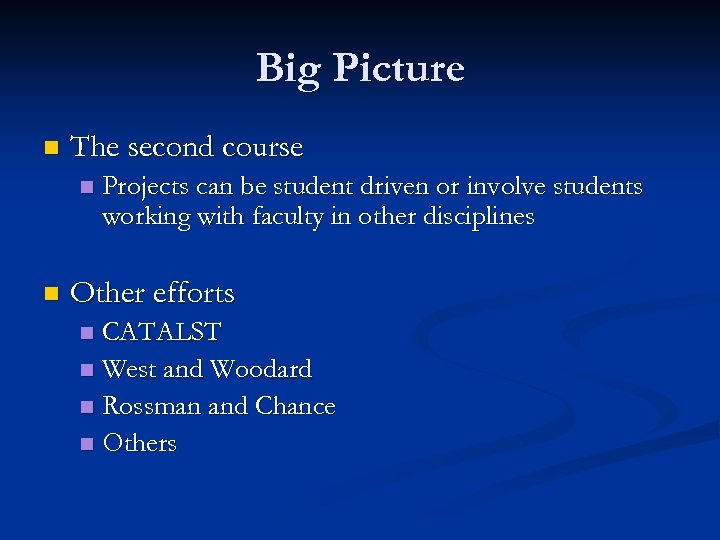Big Picture n The second course n n Projects can be student driven or involve students working with faculty in other disciplines Other efforts CATALST n West and Woodard n Rossman and Chance n Others n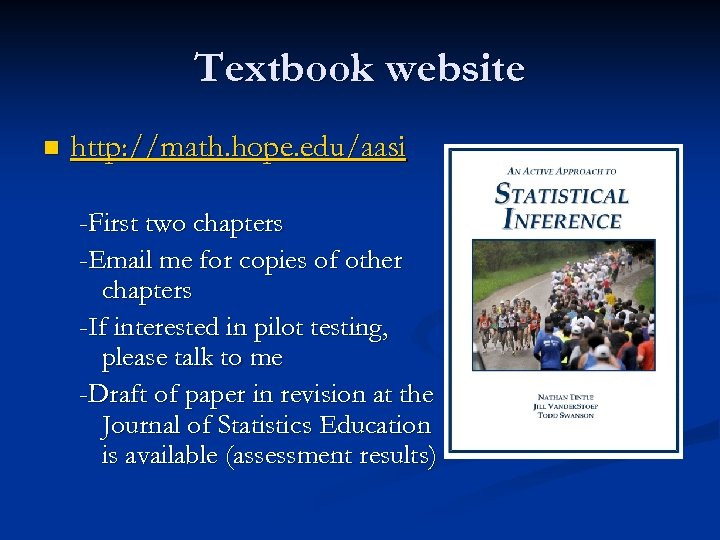Textbook website n http: //math. hope. edu/aasi -First two chapters -Email me for copies of other chapters -If interested in pilot testing, please talk to me -Draft of paper in revision at the Journal of Statistics Education is available (assessment results)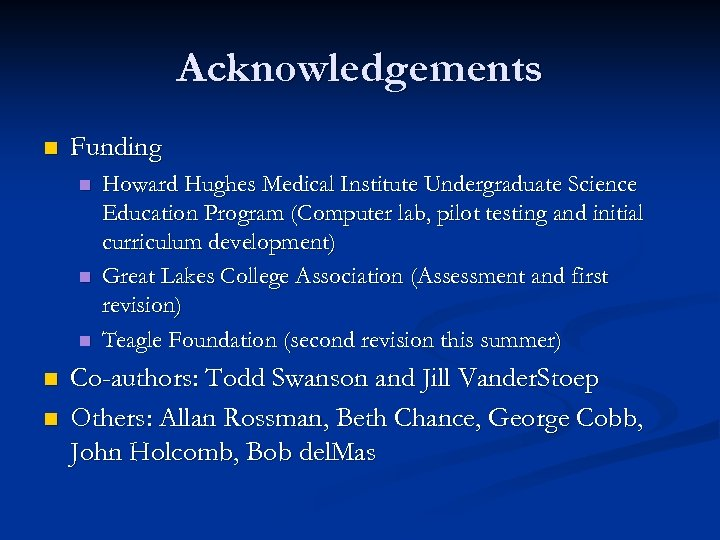Acknowledgements n Funding n n n Howard Hughes Medical Institute Undergraduate Science Education Program (Computer lab, pilot testing and initial curriculum development) Great Lakes College Association (Assessment and first revision) Teagle Foundation (second revision this summer) Co-authors: Todd Swanson and Jill Vander. Stoep Others: Allan Rossman, Beth Chance, George Cobb, John Holcomb, Bob del. Mas